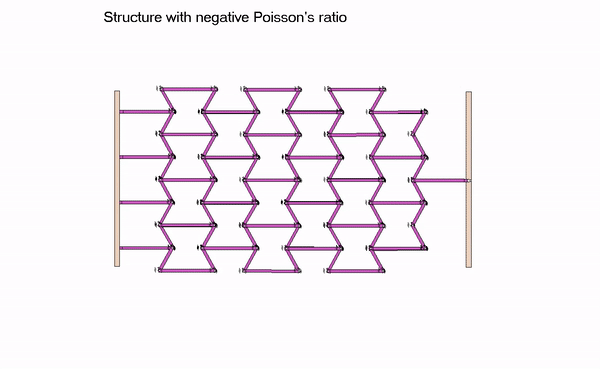# Poisson's ratio• Tensile/compressive stress in the x-direction produces tensile/compressive strain in the x-direction, with the stress and strain related through Young's modulus E:
• Strain in the x-direction produces strains the y- and z-directions. The strains in the y- and z-directions are proportional to the strain in the x-direction through the negative of the Poisson's ratio.

Positive Poisson's ratio
If the Poisson's ratio of the material if positive, then tensile/compressive strains in the x-direction produce compressive/tensile strains in the y- and z-directions, as shown below.Negative Poisson's ratio
If the Poisson's ratio of the material if positive, then tensile/compressive strains in the x-direction produce tensile/compressive strains in the y- and z-directions, just the opposite of that for a positive Poisson's ratio.

QUESTION: Can a material possess a negative Poisson’s ratio? The answer is “yes”. Such materials are known as “auxetic materials”. From above, we see than an auxetic material will expand in the transverse directions for a tensile axial load. Consider the animation below of such a material, and study how a negative value for Poisson's ratio is possible with this material. As can be seen here, a compressive axial load produces contraction in the transverse direction, and a tensile axial load produces expansion in the transverse direction.There are a number of types of polyurethane foam materials with cell structures resembling the structure shown above. Kevlar woven composite materials used for body armor are also auxetic materials.